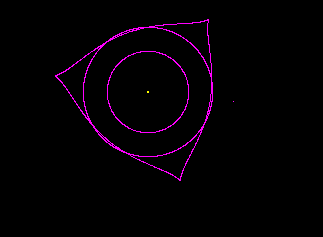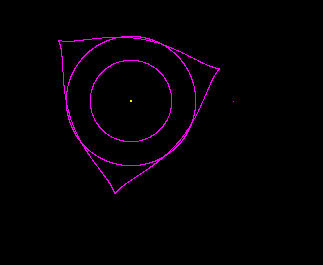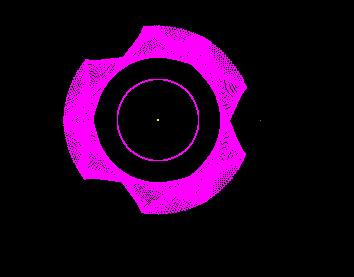# Orbit Stability Question

I was looking at some interesting resonant orbits in our solar system and was wondering if someone who knows a lot more about planetary orbits than I might be able to answer if a certain scenario would actually lead to stable orbits or not. The scenario I have in mind is four planets tightly packed around their primary so that the first, second, and fourth planets are in a 4:2:1 orbital resonance like Io, Europa, and Ganymede are. Then the third planet from the primary (probably smaller than the others) is placed in a slightly eccentric orbit (I'm thinking eccentricity between .15 and .2) between the second and fourth so that it is in a 3:2 orbital resonance with the fourth planet, and therefore a 3:4 orbital resonance with the second. This could put it in an orbit similar to how the Hilda family of asteroids are to Jupiter, so that whenever it approaches the orbit of the second planet or the fourth planet, the other planet is well ahead, behind, or on the far side of their orbit.
I know all the pieces are stable as we have real life examples, but put together I don't know what would happen. Over all, they would be in a 8:4:3:2 orbital resonance.

I am also interested in this question, but I got confused with the ratio of number of orbits completed in the same time interval, and the ratio of orbital periods (which would be the inverse ratio).
with Galilean moons it is easy, they can be written symmetrically 1:2:4 (ratio of orbital periods) or 4:2:1 (ratio of number of orbits)

But taking your case with 8:4:3:2 as ratio of number of orbits
if we convert it to ratio of orbital periods, we get:
1: 2: 8/3: 4

And since I just wanted to ask the same question but using the ratio of orbital periods so they will be
1:2:3:4

clearly this two are different ratios, although they both have representation that satisfies the 3:4 and 2:3 rules at one of the options.
which is the correct way? It is not 100% clear.

Regards,

Qshadow, I have done a bit of research on the subject and by far the most common way I have seen to write orbital resonance is the ratio of number of orbits completed in the same time interval. You could easily state it either way, I just went with what I've seen done more often. Yes, stated as ratio of orbital periods it would be (going from inner to outer) 1:2:8/3:4, or 3:6:8:12 if we want to stick to whole numbers.

tony873004
Gold Member
http://orbitsimulator.com/gravitySimulatorCloud/pfq.html
Here's a sim of this scenario. All planets are 1 Earth mass in circular orbits except #3. It has an ecc of 0.2 and a mass of 0.5 Earth masses. They orbit a sun-mass star with periods of: 10 days, 20 days, 80/3 days, and 40 days. From closest to farthest, each planet begins 60 degrees ahead of the previous one.
The bottom image shows a rotating frame holding the 4th planet stationary. It seems to be stable at least initially. See what happens if you let it run deep into the future.

tony873004, thanks for that. Initially I was wondering how useful that specific simulation would be since the orbit of the third planet was not at the angle I wanted with the second and fourth planet. I wanted it to start so that with respect to the outer planet's orbit, the third planet would trace close to a triangle with the fourth planet in the middle of the base. Like this:But your simulation started with that triangle tilted, like so:But as I watched the simulation, I noticed that the orbit of the third planet changed, and the "triangle" traced by the third planet rotated until it was exactly where I wanted it to start:Then began oscillating back and forth around that point. So that shows me that for this simulation at least, that orbit would seem to be very stable. After a thousand orbits or so, the orbits look exactly the same and this is the path the third planet has traced out with respect to the fourth. To me, this suggests pretty good orbital stability.I have done a bit of research on the subject and by far the most common way I have seen to write orbital resonance is the ratio of number of orbits completed in the same time interval.
Thanks for clarification.

And after seeing that nice simulation i wonder if we can generalize this law further, e.g. probably it work for orbit ratios that are powers of two:
32:16:8:4:2:1

But what about packing as much satellites as possible using the rule that we saw now, eg:
32:24:16:12:8:6:4:3:2

so each number between the powers of two is the sum of powers of two at (n-1) + (n-2)
would it be stable as well?